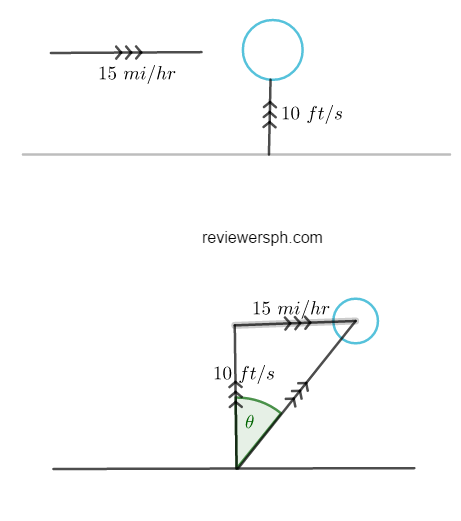### Math Notes

Subjects

#### Trigonometry Solutions

##### Topics || Problems

A balloon is rising at the rate of 10 ft a second and is being carried horizontally by a wind which has a velocity of 15 miles an hour. Find the actual velocity and the angle that its path makes with the vertical.$$15~mi/hr$$ can be converted as $$22 ~ft /s$$

The actual velocity ,$$v$$ of the wind is the hypotenuse of the triangle.

Thus, $$v^2 = 10^2+22^2$$

$$v = \sqrt{10^2+22^2}$$

$$v =24.17~ft/sec$$

The value of $$\theta$$ can be calculated using $$\tan$$.

Thus:

$$\tan \theta = \frac{22}{10}$$

$$\theta = \arctan {\frac{22}{10}}$$

$$\theta = 65.55^o$$